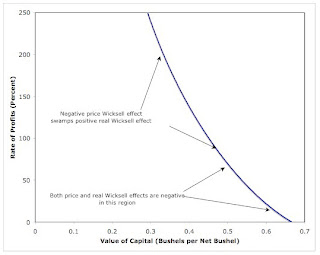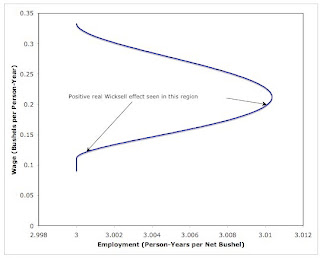## Wednesday, December 09, 2009

### Negative Price Wicksell Effect, Positive Real Wicksell Effect

1.0 Introduction
I have previously suggested a taxonomy of Wicksell effects. This post presents an example with:
• The cost-minimizing technique varying continuously along the so-called factor-price frontier
• Negative price Wicksell effects
• Positive real Wicksell effects
• Price Wicksell effects greater in magnitude than real Wicksell effects.
This example is due to Saverio M. Fratini ("Reswitching and Decreasing Demand for Capital").

2.0 Technology
Suppose technology consists of a continuum of techniques indexed by the parameter θ, where θ is a real number restricted to the interval [0, 1]:
0 ≤ θ ≤ 1
Each technique consists of the three Constant-Returns-to-Scale processes in Table 1. No commodity is basic, in Sraffa's sense, in any technique in this technology. In the first process in a technique, θ-grade iron is produced directly from unassisted labor. In the second process, labor transforms the θ-grade iron into θ-grade steel. Finally, in the third process, labor transforms θ-grade steel into corn, the consumption good in the model. All processes take a year to complete, and all processes totally use up their input.
 Inputs Industry Sector θ-GradeIron θ-GradeSteel Corn Labor (Person-Yrs) 1/(1 + θ) θ 3/(1 + θ) Iron (Tons) 0 1 0 Steel (Tons) 0 0 1 Corn (Bushels) 0 0 0 Output 1 Ton 1 Ton 1 Bushel
Capital goods are specific in their uses in this example. θ1-grade steel cannot be made out of θ2-grade iron when θ1 ≠ θ2.

3.0 Stationary-State Quantity Flows
Suppose in Table 1 that:
• The first process is used to produce (1 + θ)/(4 + θ + θ2) tons of θ-grade iron
• The second process is used to produce (1 + θ)/(4 + θ + θ2) tons of θ-grade steel
• The third process is used to produce (1 + θ)/(4 + θ + θ2) bushels corn
Then one person-year would be employed over these three processes. Capital goods would consist of (1 + θ)/(4 + θ + θ2) tons of θ-grade iron and (1 + θ)/(4 + θ + θ2) tons of θ-grade steel. The capital goods would be used up throughout the latter two sectors, but reproduced at the end of the year. Net output would consist of (1 + θ)/(4 + θ + θ2) bushels corn per person-year.

4.0 Prices
Given the technique, stationary state prices must satisfy the following three equations:
[1/(1 + θ)] w = p1
p1(1 + r) + θ w = p2
p2(1 + r) + [3/(1 + θ)] w = 1
where:
• p1 is the price of a ton θ-grade iron;
• p2 is the price of a ton θ-grade steel;
• w is the wage;
• r is the rate of profits.
A bushel corn is the numeraire. The above equations embody the assumption that labor is paid at the end of the year.

The above is a system of three equations in four unknowns, given the technique. It is a linear system, given the rate of profits. The solution in terms of the rate of profits is easily found. The so-called factor-price curve for a technique is:
w(r, θ) = (1 + θ)/[3 + θ(1 + θ)(1 + r) + (1 + r)2]
The price of a ton θ-grade iron is:
p1(r, θ) = 1/[3 + θ(1 + θ)(1 + r) + (1 + r)2]
The price of a ton θ-grade steel is:
p2(r, θ) = [(1 + r) + θ(1 + θ)]/[3 + θ(1 + θ)(1 + r) + (1 + r)2]
Given the technique and the rate of profits, these prices can be used to evaluate the value of the capital goods used in a stationary state.

5.0 The Cost-Minimizing Technique
The optimal technique to use at any given rate of profits maximizes the wage. The first-order condition for such maximization is found by equating the derivative of the factor-price curve to zero:
dw/dθ = 0
Or:
3 + θ(1 + θ)(1 + r) + (1 + r)2 - (1 + θ)(1 + 2θ)(1 + r) = 0
For 0 ≤ r ≤ 2, the cost-minimizing technique is then:
θ(r) = {[3 + (1 + r)2]/(1 + r)}1/2 - 1
For r > 2, a corner solution is found:
θ(r) = 1
Figure 1 illustrates the cost-minimizing technique.Figure 1: The Choice of Technique
The graph in Figure 1 reaches a minimum at a rate of profits of (31/2 - 1). For (121/4 - 1) < θ < 1, two rate of profits have the corresponding cost-minimizing technique indexed by the given value of θ. In other words, this is an example of reswitching.

The index for the cost-minimizing technique can be plugged into the factor price curve for the technique to which it corresponds at a given rate of profits. Figure 2 displays the resulting so-called factor price frontier. The index θ varies continuously for 0 ≤ r ≤ 200% in Figure 2. As the rate of profits increases without bound, the frontier approaches a wage of zero.Figure 2: The Factor-Price Frontier

6.0 Capital and Labor "Markets"
Fratini’s notes that this is a reswitching example in which the capital market initially appears to be in accord with out-dated neoclassical intuition. The above analysis has shown how to find physical quantities of capital goods per worker, how to evaluate them at equilibrium prices, and how to find net output per worker. Figure 3 shows the resulting plot of the value of capital per unit output. Fratini looks at the value of capital per worker instead. Either curve is continuous and downward-sloping. The regions above and below the rate of profits of (31/2 - 1) appear qualitatively similar and visually indistinguishable. This curve might be said to be a downward-sloping demand function for capital.Figure 3: The Capital Market
The analogous curve looks very different for the labor market (Figure 4). The region with a positive Wicksell effect is a region with a high rate of profits and thus a low real wage. The demand function for labor might be said to be upward-sloping in the region with a positive real Wicksell effect.Figure 4: The Labor Market

7.0 Conclusion
The example makes Fratini’s point. The shape of the relationship between the value of capital, either per worker or per unit output, and the rate of profits is not necessarily a good indicator of the presence of reswitching or reverse capital-deepening.